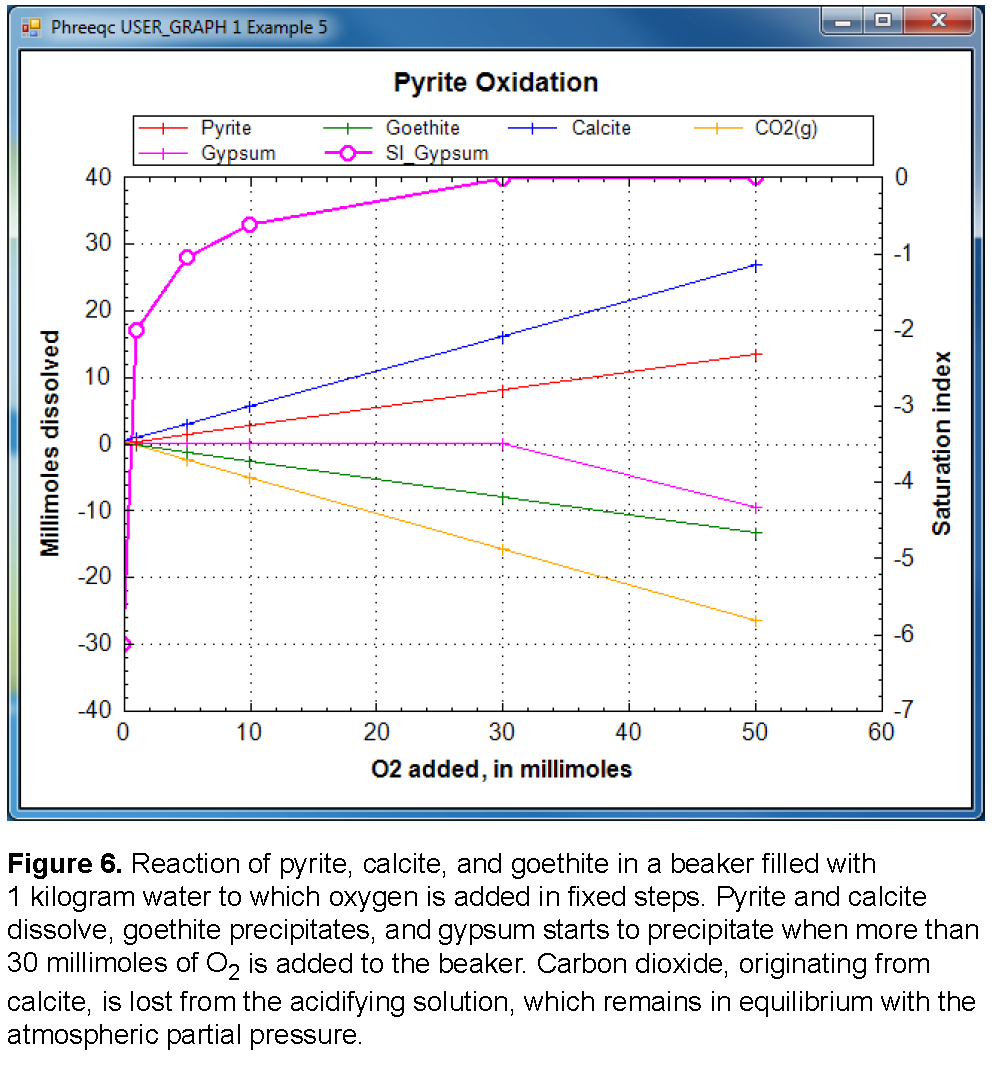| Next || Previous || Top |

## Example 5--Irreversible Reactions

This example demonstrates the irreversible reaction capabilities of PHREEQC in modeling the oxidation of pyrite. Oxygen (O 2 ) and NaCl are added irreversibly to pure water in six amounts (0.0, 0.001, 0.005, 0.01, 0.03, and 0.05 mol); the relative proportion of O 2 to NaCl in the irreversible reaction is 1.0 to 0.5. Pyrite, calcite, and goethite are allowed to dissolve to equilibrium and the carbon dioxide partial pressure is maintained at 10 -3.5 (atmospheric partial pressure). In addition, gypsum is allowed to precipitate if it becomes supersaturated.

Pure water is defined with SOLUTION input (table 19), and the pure-phase assemblage is defined with EQUILIBRIUM_PHASES input. By default, 10 mol of pyrite, goethite, calcite, and carbon dioxide are present in the pure-phase assemblage, but gypsum is defined to have 0.0 mol in the pure-phase assemblage. Gypsum can precipitate if it becomes supersaturated; it cannot dissolve initially because no moles are present. The REACTION data block defines the irreversible reaction that is to be modeled. In this example, oxygen (“O2”) will be added with a relative coefficient of 1.0 and NaCl will be added with a relative coefficient of 0.5. The steps of the reaction are defined to be 0.0, 0.001, 0.005, 0.01, 0.03, and 0.05 mol. The reactants can be defined by a chemical formula, as in this case (“O2” and “NaCl”) or by a phase name that has been defined with PHASES input. Thus, the phase names “O2(g)” or “Halite” from the default database file could have been used in place of “O2” or “NaCl” to achieve the same result. The number of moles of oxygen atoms added is equal to the stoichiometric coefficient of oxygen in the formula “O2” (2.0) times the relative coefficient (1.0) times the moles of reaction defined by the reaction step (0.0, 0.001, 0.005, 0.01, 0.03 or 0.05). Thus, in the last step, 2.0 × 1.0 × 0.05 = 0.1 mol of O atoms are added. Similarly, the number of moles of chloride added at each step is the stoichiometric coefficient of chlorine in the formula “NaCl” (1.0) times the relative coefficient (0.5) times the moles in the reaction step. SELECTED_OUTPUT and USER_GRAPH are used to write the total concentration of chloride, the saturation index of gypsum, and the total amounts and mole transfers of pyrite, goethite, calcite, carbon dioxide, and gypsum to the file ex5.sel and to plot the reactions in the chart after each equilibrium calculation.

 `TITLE Example 5.--Add oxygen, equilibrate with pyrite, calcite, and goethite.` `SOLUTION 1 PURE WATER` ` pH 7.0` ` temp 25.0` `EQUILIBRIUM_PHASES 1` ` Pyrite 0.0` ` Goethite 0.0` ` Calcite 0.0` ` CO2(g) -3.5` ` Gypsum 0.0 0.0` `REACTION 1` ` O2 1.0` ` NaCl 0.5` ` 0.0 0.001 0.005 0.01 0.03 0.05` `SELECTED_OUTPUT` ` -file ex5.sel` ` -total Cl` ` -si Gypsum` ` -equilibrium_phases pyrite goethite calcite CO2(g) gypsum` `USER_GRAPH Example 5` ` -headings Pyrite Goethite Calcite CO2(g) Gypsum SI_Gypsum` ` -chart_title "Pyrite Oxidation"` ` -axis_titles "O2 added, in millimoles" "Millimoles dissolved" \` ` "Saturation index"` ` 10 x = RXN * 1e3` ` 20 PLOT_XY x, 1e3 * (10 - EQUI("Pyrite")), symbol = Plus` ` 30 PLOT_XY x, 1e3 * (10 - EQUI("Goethite")), symbol = Plus` ` 40 PLOT_XY x, 1e3 * (10 - EQUI("Calcite")), symbol = Plus` ` 50 PLOT_XY x, 1e3 * (10 - EQUI("CO2(g)")), symbol = Plus` ` 60 PLOT_XY x, 1e3 * (-EQUI("Gypsum")), symbol = Plus, color = Magenta` ` 70 PLOT_XY x, SI("Gypsum"), y-axis = 2, line_width = 2, symbol = Circle, \` ` symbol_size = 8, color = Magenta` `END`

The results for example 5 are summarized in table 20 and displayed in figure 6. When no oxygen or sodium chloride is added to the system, a small amount of calcite and carbon dioxide dissolves, and trace amounts of pyrite and goethite react; the pH is 8.27, the pe is low (-4.94) because of equilibrium with pyrite, and gypsum is six orders of magnitude undersaturated (saturation index -6.13). As oxygen and sodium chloride are added, pyrite oxidizes, and goethite, being relatively insoluble, precipitates. This reaction generates sulfuric acid, decreases the pH, slightly increases the pe, and causes calcite to dissolve and carbon dioxide to be released. When slightly more than 30 mmol of oxygen is added, gypsum reaches saturation and begins to precipitate. When 50 mmol of oxygen and 25 mmol of sodium chloride have been added, a total of 9.55 mmol of gypsum has precipitated.

Table 20. Selected results for example 5.

[Mole transfer is relative to the moles in the phase assemblage. Positive numbers indicate an increase in the amount of the phase present; that is, precipitation. Negative numbers indicate a decrease in the amount of the phase; that is, dissolution]

pH

pe

Mole transfer, millimoles

Saturation

index of

gypsum

O 2

NaCl

Pyrite

Goethite

Calcite

CO 2(g)

Gypsum

• 0.0
• 0.0
• 8.27
• 0.00001
• -0.50
• -0.49
• 0.0
• -6.13
• 1.0
• 0.5
• 8.17
• 0.27
• -0.93
• 0.14
• 0.0
• -2.01
• 5.0
• 2.5
• 7.98
• 1.33
• -2.94
• 2.39
• 0.0
• -1.05
• 10.0
• 5.0
• 7.88
• 2.67
• -5.56
• 5.10
• 0.0
• -0.64
• 30.0
• 15.0
• 7.72
• 8.00
• -16.18
• 15.82
• 0.0
• -0.01
• 50.0
• 25.0
• 7.72
###### -13.33
• 13.33
• -26.84
• 26.49
• 9.55
• 0.00| Next || Previous || Top |